Latest Banking jobs   »

# Reasoning Ability Quiz For IDBI AM/Executive 2022- 7th June

Directions (1-5): A number arrangement machine, when given a particular input of numbers, rearranges them following a particular rule. The following is the illustration of the input and the steps of arrangement.

Input: 21, 38, 18, 20, 56, 72, 48, 44, 76, 82, 60, 32, 28, 94
Step I: 20, 33, 11, 18, 55, 67, 44, 44, 75, 76, 54, 31, 22, 89
Step II: 22, 32, 10, 20, 54, 66, 46, 46, 74, 78, 56, 30, 24, 88
Step III: 9, 14, 3, 8, 25, 31, 21, 21, 35, 37, 26, 13, 10, 42
Step IV: 18, 42, 6, 24, 50, 62, 42, 42, 70, 74, 78, 26, 30, 126
Step V: 9, 36, 6, 18, 45, 54, 36, 36, 63, 63, 63, 18, 27, 117
Step V is the last step of the above arrangement.
As per the rules followed in the above steps, find out the answer of each of the following question based on the given input.

32, 25, 62, 34, 83, 65, 73, 92, 36, 41, 89, 46, 30, 52

Reasoning Ability Quiz For IDBI AM/Executive 2022 7th June CHECK IN HINDI

Q1. Which element is fifth from the right end in step III?
(a) 32
(b) 114
(c) 42
(d) 18
(e) None of these

Q2. Which of the following operation is done in step IV of the given input output?
(a) Numbers are multiplied by 2
(b) Sum of the digits are subtracted from the number
(c) Odd numbers are multiplied by 2 and even numbers by 3
(d) Numbers are divided by sum of their digits
(e) None of these

Q3. Which of the following property is common in the elements present in step V?
(a) All numbers are odd numbers
(b) Each number is divisible by 4
(c) Each number is divisible by 2
(d) Numbers are divisible by 9
(e) Both (b) and (d)

Q4. How many prime numbers are there in step III of the given input?
(a) Three
(b) Four
(c) Seven
(d) Five
(e) Nine

Q5. Which of the following would be step II of the arrangement?
(a) 30, 24, 60, 32, 80, 66, 68, 84, 32, 40, 90, 46, 26, 48
(b) 26, 30, 84, 42, 114, 62, 96, 120, 42, 54, 86, 42, 22, 66
(c) 34, 84, 30, 42, 63, 119, 96, 120, 42, 54, 86, 42, 22, 66
(d) 31, 22, 58, 33, 71, 64, 69, 85, 35, 38, 88, 44, 27, 49
(e) 35, 22, 58, 33, 74, 64, 69, 85, 33, 33, 88, 44, 27, 49

Directions (6-10): A number arrangement machine when given an input line of numbers rearranges them following a particular rule in each step. The following is an illustration of input and rearrangement.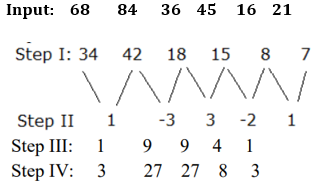Step V is the last step of the above arrangement.
As per rules followed in the above steps, find out in each of the given questions the appropriate steps for the given input.

Input – 78  48  56  87  57  39

Q6. What will be the sum of numbers present in step II?
(a) 13
(b) 14
(c) 8
(d) 11
(e) None of the above

Q7. Which element is 4th from the right end in step IV of the given input?
(a) 32
(b) 76
(c) 72
(d) 3
(e) None of these

Q8. Which among the following is the last element (from the left end) in step III of the given input?
(a) 16
(b) 36
(c) 18
(d) 1
(e) None of these

Q9. Which of the following would be the Step I?
(a) 39 24 28 29 19 13
(b) 24 39 28 29 13 19
(c) 39 28 24 29 19 13
(d) 24 28 39 19 13 24
(e) None of these

Q10. What will be the sum of numbers present in step IV?
(a) 184
(b) 176
(c) 182
(d) 181
(e) None of these

Directions (11-15): An input-output is given in different steps. Some mathematical operations are done in each step. No mathematical operation is repeated in next step.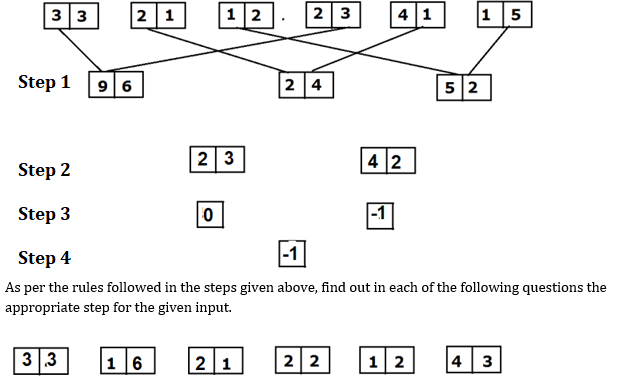Q11. Which of the following combinations will come in step III of the given input?
(a) 3, -2
(b) -2, 4
(c) 2, -1
(d) 5, 0
(e) 2, 8

Q12. Find the addition of numbers obtained in Step II?
(a) 45
(b) 48
(c) 105
(d) 57
(e) 65

Q13. Find the difference between the two numbers obtained in Step III?
(a) 3
(b) 4
(c) -3
(d) 2
(e) 5

Q14. If the last step number is multiplied by 4 what will be the final answer?
(a) 15
(b) 20
(c) -16
(d) 12
(e) None of the above

Q15. If all the digits of each block in step I are added, then what will be the answer?
(a) 26
(b) 30
(c) 28
(d) 32
(e) None of the above

Solutions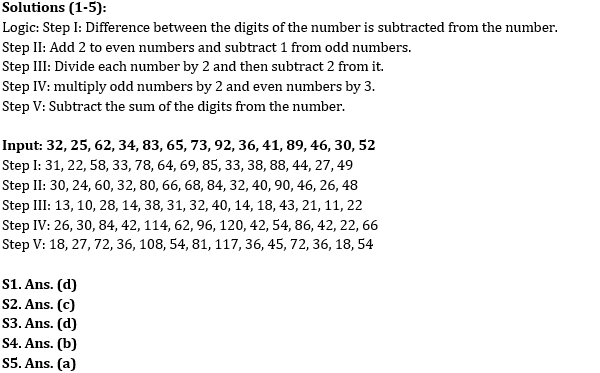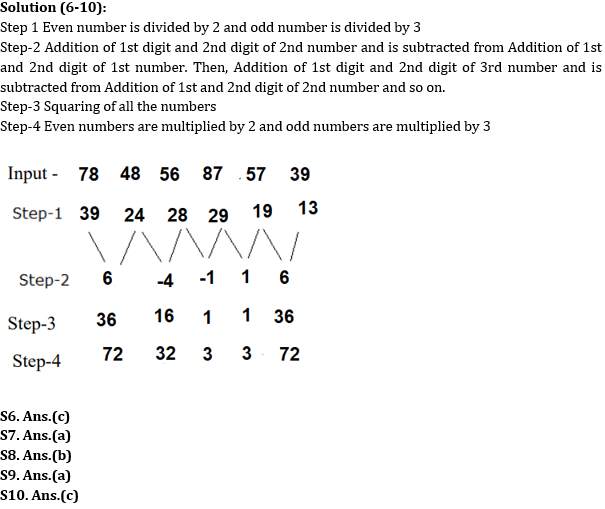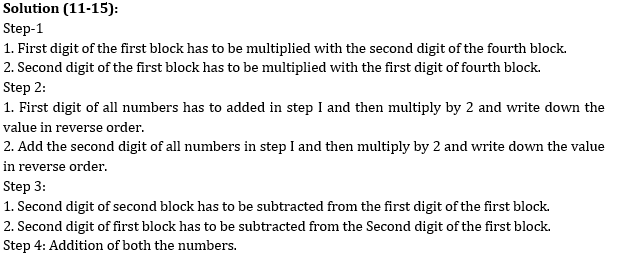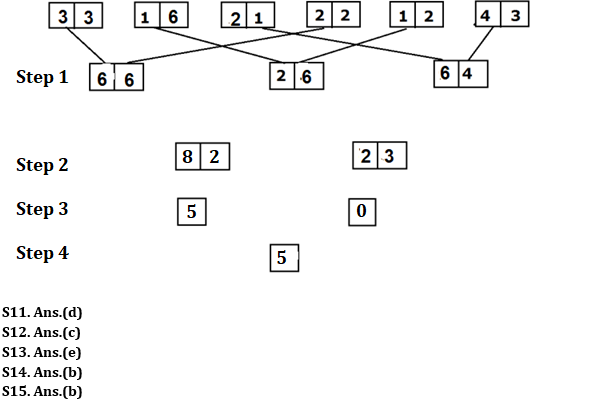#### Congratulations!